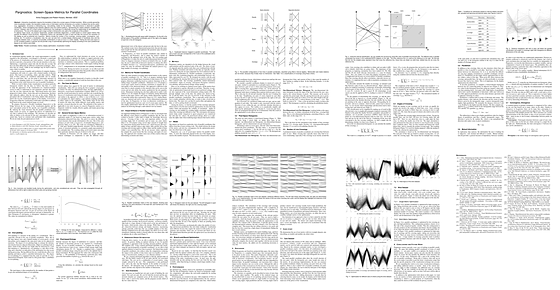# Pargnostics: Screen-Space Metrics for Parallel Coordinates ​Interactive visualization requires the translation of data into a screen space of limited resolution. While currently ignored by most visualization models, this translation entails a loss of information and the introduction of a number of artifacts that can be useful, (e.g., aggregation, structures) or distracting (e.g., over-plotting, clutter) for the analysis. This phenomenon is observed in parallel coordinates, where overlapping lines between adjacent axes form distinct patterns, representing the relation between variables they connect. However, even for a small number of dimensions, the challenge is to effectively convey the relationships for all combinations of dimensions. The size of the dataset and a large number of dimensions only add to the complexity of this problem. To address these issues, we propose Pargnostics, parallel coordinates diagnostics, a model based on screen-space metrics that quantify the different visual structures. Pargnostics metrics are calculated for pairs of axes and take into account the resolution of the display as well as potential axis inversions. Metrics include the number of line crossings, crossing angles, convergence, over-plotting, etc. To construct a visualization view, the user can pick from a ranked display showing pairs of coordinate axes and the structures between them, or examine all possible combinations of axes at once in a matrix display. Picking the best axes layout is an NP-complete problem in general, but we provide a way of automatically optimizing the display according to the user’s preferences based on our metrics and model.

Aritra Dasgupta and Robert Kosara, Pargnostics: Screen-Space Metrics for Parallel Coordinates, Transactions on Visualization and Computer Graphics (Proceedings InfoVis), vol. 16, no. 6, pp. 1017–1026, 2010. DOI: 10.1109/TVCG.2010.184

bibtex
``````@article{Dasgupta:InfoVis:2010,
year = 2010,
title = {Pargnostics: Screen-Space Metrics for Parallel Coordinates},
author = {Aritra Dasgupta and Robert Kosara},
venue = {Transactions on Visualization and Computer Graphics (Proceedings InfoVis)},
volume = {16},
number = {6},
pages = {1017–1026},
doi = {10.1109/TVCG.2010.184},
acceptance-rate = {26},
abstract = {Interactive visualization requires the translation of data into a screen space of limited resolution. While currently ignored by most visualization models, this translation entails a loss of information and the introduction of a number of artifacts that can be useful, (e.g., aggregation, structures) or distracting (e.g., over-plotting, clutter) for the analysis. This phenomenon is observed in parallel coordinates, where overlapping lines between adjacent axes form distinct patterns, representing the relation between variables they connect. However, even for a small number of dimensions, the challenge is to effectively convey the relationships for all combinations of dimensions. The size of the dataset and a large number of dimensions only add to the complexity of this problem. To address these issues, we propose Pargnostics, parallel coordinates diagnostics, a model based on screen-space metrics that quantify the different visual structures. Pargnostics metrics are calculated for pairs of axes and take into account the resolution of the display as well as potential axis inversions. Metrics include the number of line crossings, crossing angles, convergence, over-plotting, etc. To construct a visualization view, the user can pick from a ranked display showing pairs of coordinate axes and the structures between them, or examine all possible combinations of axes at once in a matrix display. Picking the best axes layout is an NP-complete problem in general, but we provide a way of automatically optimizing the display according to the user’s preferences based on our metrics and model.},
}``````
``````@article{Dasgupta:InfoVis:2010,
year = 2010,
title = {Pargnostics: Screen-Space Metrics for Parallel Coordinates},
author = {Aritra Dasgupta and Robert Kosara},
venue = {Transactions on Visualization and Computer Graphics (Proceedings InfoVis)},
volume = {16},
number = {6},
pages = {1017–1026},
doi = {10.1109/TVCG.2010.184},
acceptance-rate = {26},
abstract = {Interactive visualization requires the translation of data into a screen space of limited resolution. While currently ignored by most visualization models, this translation entails a loss of information and the introduction of a number of artifacts that can be useful, (e.g., aggregation, structures) or distracting (e.g., over-plotting, clutter) for the analysis. This phenomenon is observed in parallel coordinates, where overlapping lines between adjacent axes form distinct patterns, representing the relation between variables they connect. However, even for a small number of dimensions, the challenge is to effectively convey the relationships for all combinations of dimensions. The size of the dataset and a large number of dimensions only add to the complexity of this problem. To address these issues, we propose Pargnostics, parallel coordinates diagnostics, a model based on screen-space metrics that quantify the different visual structures. Pargnostics metrics are calculated for pairs of axes and take into account the resolution of the display as well as potential axis inversions. Metrics include the number of line crossings, crossing angles, convergence, over-plotting, etc. To construct a visualization view, the user can pick from a ranked display showing pairs of coordinate axes and the structures between them, or examine all possible combinations of axes at once in a matrix display. Picking the best axes layout is an NP-complete problem in general, but we provide a way of automatically optimizing the display according to the user’s preferences based on our metrics and model.},
}``````